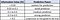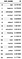# Weight of evidence and Information Value using Python

Weight of evidence (WOE) and Information value (IV) are simple, yet powerful techniques to perform variable transformation and selection. These concepts have huge connection with the logistic regression modeling technique. It is widely used in credit scoring to measure the separation of good vs bad customers.

The formula to calculate WOE and IV is provided below.

or simply,

Here is a simple table that shows how to calculate these values.

The advantages of WOE transformation are

1. Handles missing values
2. Handles outliers
3. The transformation is based on logarithmic value of distributions. This is aligned with the logistic regression output function
4. No need for dummy variables
5. By using proper binning technique, it can establish monotonic relationship (either increase or decrease) between the independent and dependent variable

Also, IV value can be used to select variables quickly.Figure3: Information value table

WOE and IV using Python

You can view the entire code in the github link.

The dataset for this demo is downloaded from UCI Machine Learning repository. Please have a look at the code, to follow the explanations provided below. A sample of the dataset is shown below.

df.head()

df.info()

I changed the ‘y’ variable to numeric and named it as ‘target’. As mentioned before, monotonic binning ensures linear relationship is established between independent and dependent variable. In the code, I have two functions mono_bin() and char_bin().The mono_bin function is used for numeric variables and char_bin is used for character variables. I used spearman correlation to perform monotonic binning.

The ‘max_bin’ variable is used to provide the maximum number of bins (categories) for numeric variable binning. For some numeric variables, the mono_bin function produce only one category while binning. To avoid that, I have another variable called ‘force_bin’ to ensure it at least produces 2 categories.

The WOE and IV calculation can be invoked using the below code.

final_iv, IV = data_vars(df , df.target)

The final_iv dataframe has all the WOE transformations and looks similar to the Figure 2 shown above. The IV dataframe gives the consolidated IV values for each input variables and looks like below.IV dataframe

Based on the above information, the variables can be selected based on their predictive power.

Now you can perform WOE variable transformation and IV variable selection using Python. Have fun!

I am passionate about Artificial Intelligence and Data Science. I focus on 360 degree customer analytics models and machine learning workflow automation.

## More from Sundar Krishnan

I am passionate about Artificial Intelligence and Data Science. I focus on 360 degree customer analytics models and machine learning workflow automation.